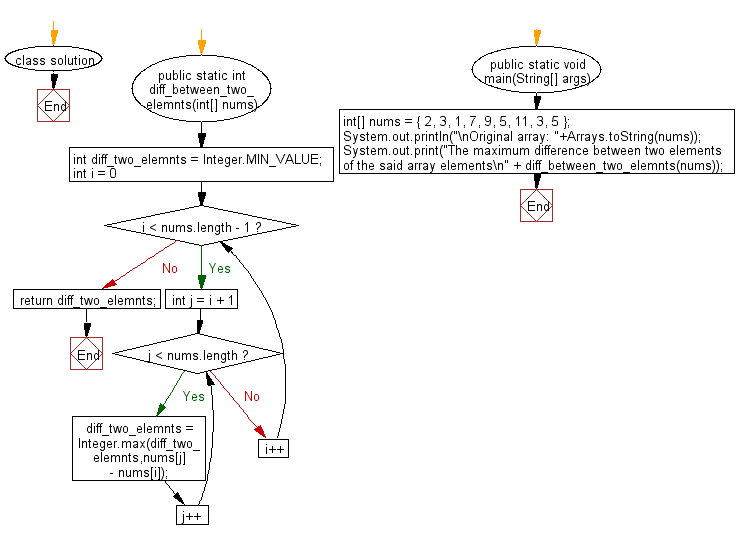﻿ Java: Maximum difference so that small elements appear first# Java Array Exercises: Find maximum difference between two elements in a given array of integers such that smaller element appears before larger element

## Java Array: Exercise-65 with Solution

Write a Java program to find maximum difference between two elements in a given array of integers such that smaller element appears before larger element.

Example:
Input :
nums = { 2, 3, 1, 7, 9, 5, 11, 3, 5 }
Output:
The maximum difference between two elements of the said array elements
10

Sample Solution:

Java Code:

``````import java.util.Arrays;
class solution
{
public static int diff_between_two_elemnts(int[] nums)
{
int diff_two_elemnts = Integer.MIN_VALUE;

for (int i = 0; i < nums.length - 1; i++) {
for (int j = i + 1; j < nums.length; j++) {
diff_two_elemnts = Integer.max(diff_two_elemnts,nums[j] - nums[i]);
}
}

return diff_two_elemnts;
}

public static void main(String[] args)
{
int[] nums = { 2, 3, 1, 7, 9, 5, 11, 3, 5 };
System.out.println("\nOriginal array: "+Arrays.toString(nums));

System.out.print("The maximum difference between two elements of the said array elements\n" + diff_between_two_elemnts(nums));
}
}
```
```

Sample Output:

```Original array: [2, 3, 1, 7, 9, 5, 11, 3, 5]
The maximum difference between two elements of the said array elements
10
```

Flowchart:Java Code Editor:

Improve this sample solution and post your code through Disqus

What is the difficulty level of this exercise?

Test your Programming skills with w3resource's quiz.

﻿

## Java: Tips of the Day

countOccurrences

Counts the occurrences of a value in an array.

Use Arrays.stream().filter().count() to count total number of values that equals the specified value.

```public static long countOccurrences(int[] numbers, int value) {
return Arrays.stream(numbers)
.filter(number -> number == value)
.count();
}
```

Ref: https://bit.ly/3kCAgLb